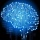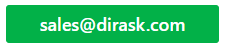Home
IT Knowledge
IT Career
Inspiration
Languages
EN

# SQL - how to search table by text in MySql / MariaDB

9 points
Created by:Root-ssh
68570

Using MySQL or MariaDB it is possible to make search query by any column in following ways.

## 1. Equal operator example

Note: to check how this example works Data base preparation section can be useful.

### 1.1. Example 1 - equal operator with exact match:

``````SELECT *
FROM `posts`
WHERE `name` = 'JavaScript';``````

Result:

### 1.2. Example 2 - equal operator with exact match:

``````SELECT *
FROM `posts`
WHERE `name` = 'JavaScript - how to access input element?';``````

Result:

### 1.3. Example 3 - few equal operators:

``````SELECT *
FROM `posts`
WHERE
`name` = 'JavaScript - how to access input element?'
AND
`content` = 'Post content 2...';``````

Result:

## 2. Like operator example

Note: to check how this example works Data base preparation section can be useful.

### 2.1. Example 1 - like operator with exact match:

``````SELECT *
FROM `posts`
WHERE `name` LIKE 'JavaScript';``````

Result:

### 2.2. Example 2 - like operator with exact left match:

``````SELECT *
FROM `posts`
WHERE `name` LIKE 'JavaScript%';``````

Result:

### 2.3. Example 3 - like operator:

``````SELECT *
FROM `posts`
WHERE `name` LIKE '%Script%';``````

Result:

### 2.4. Example 4 - few like operators:

Note: this approach can be used to find records which contain separated worlds avoiding worlds ordering.

``````SELECT *
FROM `posts`
WHERE
`name` LIKE '%Script%'
AND
`name` LIKE '%how%';``````

Result:

## 3. Regex function example

Note: to check how this example works Data base preparation section can be useful.

### 3.1. Example 1 - regex operator:

``````SELECT *
FROM `posts`
WHERE `name` REGEXP '.*Script.*';``````

Result:

### 3.2. Example 2 - regex operator with few following worlds:

``````SELECT *
FROM `posts`
WHERE `name` REGEXP '.*Script.*how.*';``````

Result:

## 4. Data base preparation

`create_tables.sql` file:

``````CREATE TABLE `posts` (
`id` INT(10) UNSIGNED NOT NULL AUTO_INCREMENT,
`name` VARCHAR(100) NOT NULL,
`content` TEXT NOT NULL,
PRIMARY KEY (`id`)
)
ENGINE=InnoDB;``````

`insert_data.sql` file:

``````INSERT INTO `posts`
(`id`, `name`, `content`)
VALUES
(1, 'jQuery - how to get value of input field?', 'Post content 1...'),
(2, 'JavaScript - how to access input element?', 'Post content 2...'),
(3, 'JavaScript - how to check type of object?', 'Post content 3...'),
(4, 'JavaScript - is variable array type?', 'Post content 4...');``````